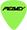Support
FREE SHIPPING ON ORDERS OVER \$20!*
0
\$0.00 0 items

No products in the cart.

# Long Speaker Cable Runs Waste Power and Reduce Performance

By Marty McCann

Recently I had a customer who had an application where he had to run a loudspeaker line 1500Ft. I suggested that he run a 70-volt distributed line instead. Since he didn't understand 70-volt lines he opted to do it his way, so I did a little math regarding speaker line losses, here are the facts:

Power in Watts equals the voltage squared, divided by the resistance of the load (V x V / R):

40 x 40 = 1600 1600 / 4 ohms = 400 Watts 1600 / 8 ohms = 200 Watts

Power in Watts also equals voltage times the current (W = V x I). It is more difficult to measure the current flowing through a device; however voltage and resistance can be determined or measured with a simple inexpensive multimeter. So, if you want to know the current that is present in the circuit, you can simply measure the voltage and resistance and calculate the power from the first formula, and then use the second formula to calculate the current present in the circuit by dividing the power in watts by the voltage to find the current (I = W / V). 400 Watts divided by 40 volts equals 10 Amperes of current flow (400 / 40 = 10).

The following charts for the wire gauges are based on an amplifier producing 40 volts, which would normally result in 400 Watts of power at 10 amperes of current into a 4 ohm load, and into an 8 ohm load the same 40 volts would produce 200 Watts of power at 5 amperes of current flow. Using A.W.G. ## 18 gauge wire with 153.6 feet per ohm of resistance as an example, if you had 1500 feet of the wire, the resistance value would be 1500 divided by 153.6, which equals 9.766 ohms of resistance (1500 / 153.5 = 9.766). But that is only the resistance of one of the two conductors in the speaker cable, in other words there is 9.766 ohms of resistance in each wire running to the speaker, or a total of 19.53 ohms of resistance in both conductors (9.766 x 2 = 19.53).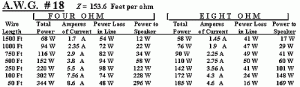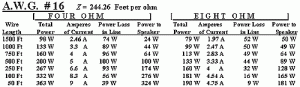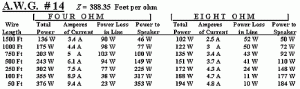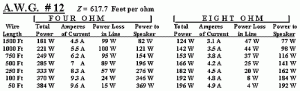The two conductors are essentially in series with the resistance of the loudspeaker. So in the case of a 4 ohm speaker and 1500 feet of ## 18 gauge wire, there is 23.53 ohms of resistance in the circuit that the power amplifier would be driving (19.53 + 4 + 23.53). Now if that amplifier if putting out 40 volts, the power produced in the circuit is 67.99 Watts (40 x 40 =1600 1600 / 23.53 = 67.99). If we round that off to 68 Watts, the current in the circuit would be determined by dividing the power in watts by the voltage, which would equal 1.7 amperes of current flow (68 / 40 = 1.7).

Using another version of ohms' law, if you know the resistance and the current, you can calculate the power dropped across a resistance by multiplying the voltage times the current squared (R x I x I). The power that actually reaches the loudspeaker would then be 1.7 times 1.7, times 4, or 11.56 Watts (1.7 x 1.7 x 4 = 11.56). Rounding off, we would have about 12 watts actually getting to the loudspeaker. The amount of power lost, or consumed by the cable would then be the total power produced by the amplifier, minus the power that reached the loudspeaker, or 54 Watts (68 ? 12 = 54). I hope I haven't hurt anyone's brain here with the 4th grade math, but I needed to show how the calculations were derived. The rule of thumb for professional sound reinforcement is to use the shortest possible loudspeaker run and a heavy gauge wire. Check out the wire chart that follows, then read on to learn more about the consequences of using too small of a wire gauge.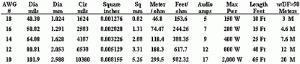# Become a Peavey Member

Get exclusive deals, benefits and access.